Navigate back to the homepage

Instanced Grass onto a gltf Model at Certain Elevation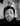Rick
November 28th, 2021 · 1 min read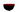The original jsfiddle came from here. This is a fiddle with grass on a simple plane..

This then got me thinking how can this be adapted to work with any model and have the grass appear on the top of it.

Here is a codesandbox with the code in for you to play around with (the grass lays ontop of the gltf model):

This is the main javascript file:

1import React, { useEffect, useRef, useMemo } from "react";
2import * as THREE from "three";
3
4import { OrbitControls } from "three/examples/jsm/controls/OrbitControls";
5import { MeshSurfaceSampler } from "three/examples/jsm/math/MeshSurfaceSampler";
6
7import { extend, useFrame, useThree, useLoader } from "@react-three/fiber";
8import { useGLTF } from "@react-three/drei";
10
11extend({ OrbitControls, MeshSurfaceSampler });
12
13let simpleNoise = `
14float N (vec2 st) { // https://thebookofshaders.com/10/
15 return fract( sin( dot( st.xy, vec2(12.9898,78.233 ) ) ) * 43758.5453123);
16}
17
18float smoothNoise( vec2 ip ){ // https://www.youtube.com/watch?v=zXsWftRdsvU
19 vec2 lv = fract( ip );
20 vec2 id = floor( ip );
21
22 lv = lv * lv * ( 3. - 2. * lv );
23
24 float bl = N( id );
25 float br = N( id + vec2( 1, 0 ));
26 float b = mix( bl, br, lv.x );
27
28 float tl = N( id + vec2( 0, 1 ));
29 float tr = N( id + vec2( 1, 1 ));
30 float t = mix( tl, tr, lv.x );
31
32 return mix( b, t, lv.y );
33}
34`;
35
37varying vec2 vUv;
38uniform float time;
39
40\${simpleNoise}
41
42void main() {
43
44 vUv = uv;
45 float t = time * 2.;
46
47 // VERTEX POSITION
48
49 vec4 mvPosition = vec4( position, 1.0 );
50 #ifdef USE_INSTANCING
51 mvPosition = instanceMatrix * mvPosition;
52 #endif
53
54 // DISPLACEMENT
55
56 float noise = smoothNoise(mvPosition.xz * 0.5 + vec2(0., t));
57 noise = pow(noise * 0.5 + 0.5, 2.) * 2.;
58
59 // here the displacement is made stronger on the blades tips.
60 float dispPower = 1. - cos( uv.y * 3.1416 * 0.15 );
61
62 float displacement = noise * ( 0.3 * dispPower );
63 mvPosition.z += displacement * noise;
64
65
66 vec4 modelViewPosition = modelViewMatrix * mvPosition;
67 gl_Position = projectionMatrix * modelViewPosition;
68
69}
70`;
71
73varying vec2 vUv;
74uniform sampler2D textureMap;
75
76void main() {
77
78 float alpha = texture2D(textureMap, vUv).r;
79 //If transparent, don't draw
80 if (alpha < 0.15) discard;
81
82 vec3 baseColor = vec3( 0.5, 0.0, 0.0 );
83 float clarity = ( vUv.y * 0.875 ) + 0.125;
84 gl_FragColor = vec4( baseColor * clarity, 1 );
85}
86`;
87
88const Terrain = () => {
89 const { nodes, materials } = useGLTF("/scene_1.gltf");
90 const {
91 scene,
92 camera,
93 gl: { domElement },
94 } = useThree();
95
96 const terrainRef = useRef(null);
97
99
100 const uniforms = {
101 time: {
102 value: 0,
103 },
104 textureMap: {
105 value: texture,
106 },
107 };
108
109 const grassMaterial = useMemo(
110 () =>
114 uniforms,
115 side: THREE.DoubleSide,
116 }),
117 []
118 );
119
120 const options = { bladeWidth: 0.012, bladeHeight: 0.11, joints: 5 };
121
122 const baseGeom = useMemo(
123 () =>
124 new THREE.PlaneBufferGeometry(
127 1,
128 options.joints
129 ).translate(0, options.bladeHeight / 2, 0),
130 [options]
131 );
132
133 useEffect(() => {
134 const instanceNumber = 30000;
135 const dummy = new THREE.Object3D();
136 var geometry = baseGeom;
137
138 const instancedMesh = new THREE.InstancedMesh(
139 geometry,
140 grassMaterial,
141 instanceNumber
142 );
143
144 instancedMesh.scale.x = 5.5;
145 instancedMesh.scale.y = 5.5;
146 instancedMesh.scale.z = 5.5;
147
148 if (terrainRef?.current) {
149 const sampler = new MeshSurfaceSampler(terrainRef?.current).build();
150 for (let i = 0; i < instanceNumber; i++) {
151 const tempPosition = new THREE.Vector3();
152 sampler.sample(tempPosition);
153
154 dummy.position.set(tempPosition.x, tempPosition.y, tempPosition.z);
155
156 dummy.updateMatrix();
157 if (tempPosition.y >= 0.0) {
158 instancedMesh.setMatrixAt(i, dummy.matrix);
159 }
160 }
161 }
162
164 }, [scene, grassMaterial]);
165
166 useFrame(({ clock }) => {
167 grassMaterial.uniforms.time.value = clock.getElapsedTime();
168 grassMaterial.uniformsNeedUpdate = true;
169 grassMaterial.uniforms.textureMap.value = texture;
170 });
171 return (
172 <>
173 <orbitControls args={[camera, domElement]} />
174 <group dispose={null}>
175 <mesh
176 ref={terrainRef}
177 geometry={nodes.Icosphere.geometry}
178 position={[0, 0, 0]}
179 scale={5.5}
180 >
181 <meshPhysicalMaterial color={"black"} />
182 </mesh>
183 </group>
184 <directionalLight color={"white"} intensity={1.3} />
185 </>
186 );
187};
188
189export default Terrain;

There are 2 bits which I did modifications to the original jsfiddle:

• sampling the mesh and adding a Y modifier
• use a alpha image to get shape of blade of grass
1. Sampling:
1useEffect(() => {
2 const instanceNumber = 30000;
3 const dummy = new THREE.Object3D();
4 var geometry = baseGeom;
5
6 const instancedMesh = new THREE.InstancedMesh(
7 geometry,
8 grassMaterial,
9 instanceNumber
10 );
11
12 instancedMesh.scale.x = 5.5;
13 instancedMesh.scale.y = 5.5;
14 instancedMesh.scale.z = 5.5;
15
16 if (terrainRef?.current) {
17 const sampler = new MeshSurfaceSampler(terrainRef?.current).build();
18 for (let i = 0; i < instanceNumber; i++) {
19 const tempPosition = new THREE.Vector3();
20 sampler.sample(tempPosition);
21
22 dummy.position.set(tempPosition.x, tempPosition.y, tempPosition.z);
23
24 dummy.updateMatrix();
25 if (tempPosition.y >= 0.0) {
26 instancedMesh.setMatrixAt(i, dummy.matrix);
27 }
28 }
29 }
30
32}, [scene, grassMaterial]);

This is the part where we sample the terrain. With the sampled position we only store positions for instancing if the y coordinate is above 0.0.

There are a couple of key points in the code.

Firstly we have to set the scale of the instanced mesh and this is dependent of what gltfjsx spits out, i.e. the scale below:

1<group dispose={null}>
2 <mesh
3 ref={terrainRef}
4 geometry={nodes.Icosphere.geometry}
5 position={[0, 0, 0]}
6 scale={5.5}
7 >
8 <meshPhysicalMaterial color={"black"} />
9 </mesh>
10</group>

Seciondly we have to update the matrix of the dummy vector, if we dont do this then you will get something like this: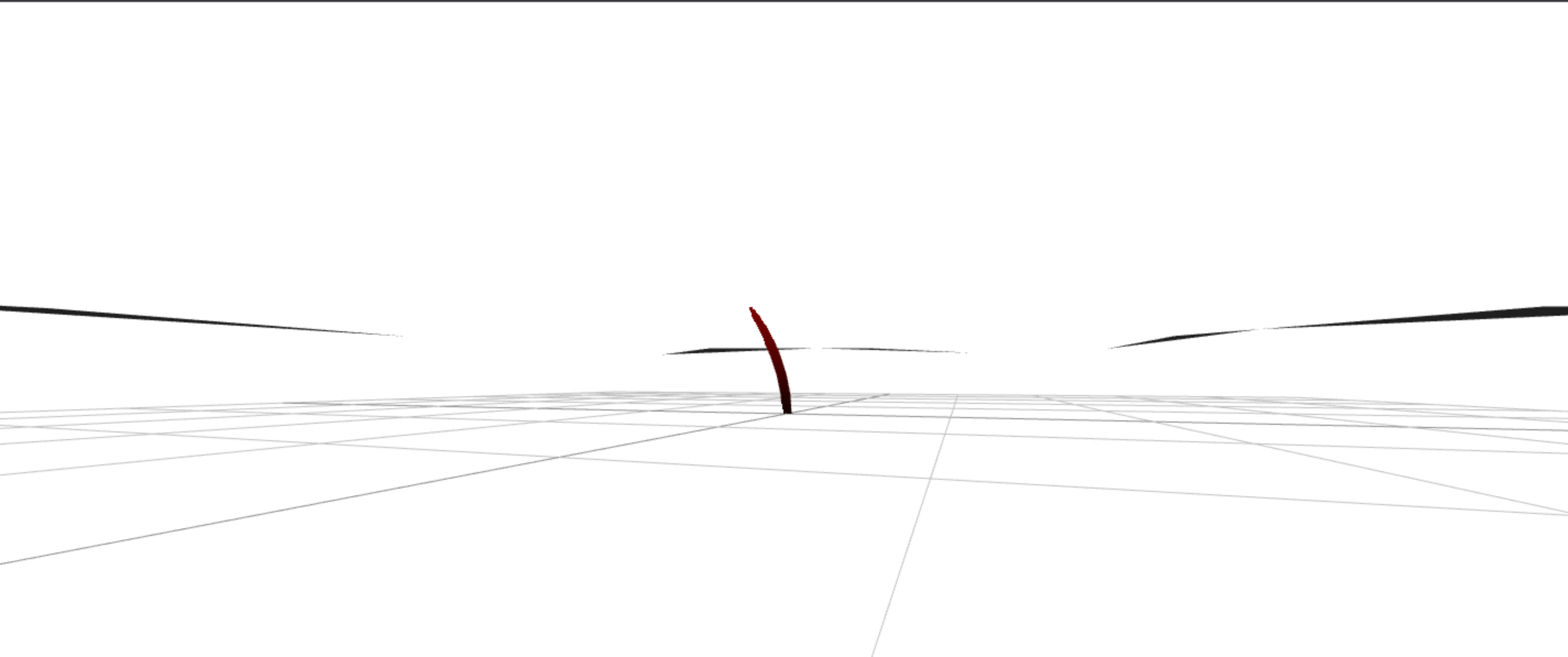This is because we are making changes to positions and scale we have to update the matrix:

1dummy.updateMatrix();
1. Alpha image for blade shape:
2 varying vec2 vUv;
3 uniform sampler2D textureMap;
4
5 void main() {
6
7 float alpha = texture2D(textureMap, vUv).r;
8
9 // If transparent, don't draw
11 if (alpha < 0.15) discard;
12
13 //.....
14 }
15`;
16//.....
17
19
20//.....
21
22useFrame(({ clock }) => {
23 //.....
24 grassMaterial.uniforms.textureMap.value = texture;
25});

The key to this is the discard key word in glsl, which essential means we dont process this fragment if the is present in the fragment shader, the result being to cut the parts of the image which have a alpha of < 0.15, ending in a blade of grass shape.

Final Thoughts:

This was a very quick and simple implementation as i modified an existing jsfidle.

The beautify of this is because we are sampling a mesh and only storing positions above a certain Y coordinate even if there are bumps in the mesh above Y = 0.0 then this will get the origin position for the blade of grass over the bumps.

Also you could carve up a large mesh to be smaller sections which have this grass.

Also as a side point you could use noise to have a gradient of grass or to create clumps of grass for a more natural effect.

Heres a video of the end results:

Until next time 😎

More articles from theFrontDev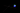Ray Marching Over a React Three Fiber (R3F) Scene

Raymarching on top of a three.js or react-three-fiber scene, leading to more advanced effects.

November 14th, 2021 · 2 min read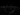How to create GPU particles in React Three Fiber (R3F)

Generating GPU particles using floatType textures, a simulation and render shader material in React Three Fiber. Using noise to add movement and changing gl_PointSize.

November 11th, 2021 · 3 min read